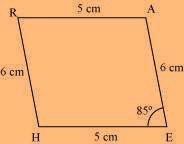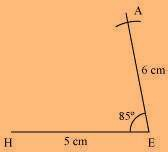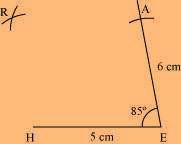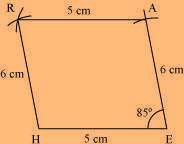# Construct the following quadrilateral: Parallelogram HEAR where HE = 5 cm, EA = 6 cm and angle R = 85o

SolutionWe can draw the quadrilateral HEAR using the steps given below

(1) Draw a line segment HE of 5 cm and an angle of 85º at point E. As vertex A is 6 cm away from vertex E, cut a line segment EA of 6 cm from this ray.(2) Vertex R is 6 cm and 5 cm away from vertex H and A respectively. Considering radii as 6 cm and 5 cm, draw arcs from points H and A respectively. These will be intersecting each other at point R.(3) Join R to H and A.(1)(0)